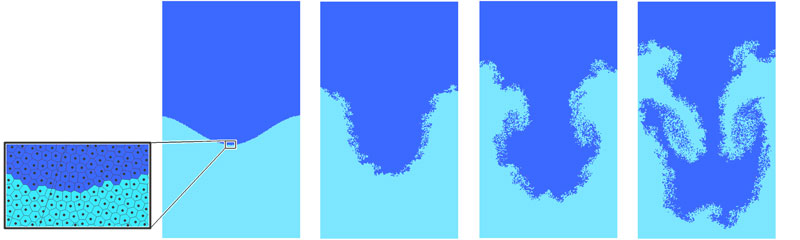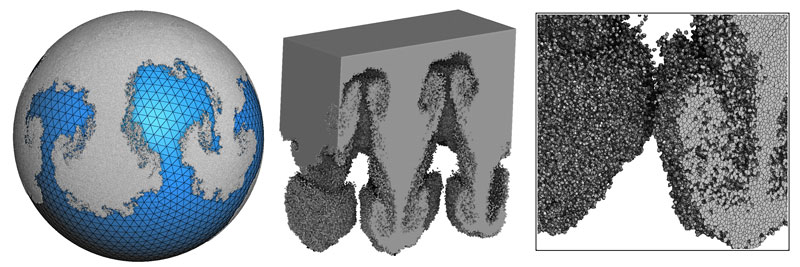# Simulating the Physics of Fluids on a Computer

by Bruno Levy (Inria)

Research teams at Inria and University Paris Sud are elaborating new mathematical methods to simulate the physics of fluids. This has the potential to improve a crucial component of digital twin technology.

Digital twins can dramatically reduce the development cost of complex systems by making it possible to test different designs in a very short time, or by simulating the behaviour of the system in various conditions. A central component in digital twin technology is the set of methods that can accurately simulate the laws of physics in a computer. As such, fluid dynamics play an important role in many different systems, from cars to spaceships, from the most sophisticated turbo-machine to (seemingly) simpler objects, such as car tires, designed to best evacuate water and optimise adherence on a wet road.

Fluids are objects that are notoriously difficult to simulate for various reasons, including both their geometries and the underlying physics:

• Shape and topology of interfaces: during its movement, a fluid can show drastic changes in shape and topology.  The free surface of a fluid can split and merge, create splashes. When considering two non-miscible fluids (water and oil), the interface between both fluids can form complicated shapes;
• Incompressibility and conservation of physical quantities: it is well known that some quantities are conserved, the most obvious one being mass. The kinetic energy is also conserved, as well as momentum, and another vector quantity that corresponds to the way things are spinning (called “angular momentum”). If considering an incompressible fluid (like most liquids), then the volume of a fluid element needs to be conserved as well. These conservation laws are not trivial to enforce in a numerical simulation.

Newton’s first law states that an isolated particle moves along a straight line with constant speed. In a fluid, the particles try to follow trajectories that are as straight as possible, but in addition they are subject to both gravity and the incompressibility constraint. The theory of things that move in as straight a line as possible while satisfying a constraint (geodesics) has for the incompressibility constraint a rich and elegant mathematical structure  that can be transformed into a computer algorithm. In this computer algorithm, the fluid is decomposed into a set of cells, with cells that follow the movement of the fluid during the simulation (Lagrangian mesh). It is different from most methods that use a fixed mesh through which the fluid flows (Eulerian mesh). This makes it easier to conserve volume and other physical quantities, and gives an explicit representation of the interfaces. It is thus possible to design a computer representation of a fluid that is by design incompressible.  In this representation, the fluid is decomposed into a set of cells (close up in Figures 1 and 2). The decomposition is parameterised by a set of points (black dots in Figure 1). Since fluids are incompressible, the cells are supposed to keep the same volume throughout the whole simulation. Enforcing this constraint is a particular instance of a well-known problem in computational mathematics (semi-discrete optimal transport ).

In cooperation with Quentin Merigot (Paris Sud University), the ALICE/GRAPHYS Inria team develops efficient computational algorithms for solving this problem . This numerical algorithm opens the door to a certain class of fluid simulation algorithms .

Figure 1 shows an example of a numerical simulation of what happens when a heavy fluid is put on top of a light fluid. Both fluids try to exchange positions, but incompressibility is a difficult obstruction. At the beginning, pairs of cells manage to exchange their positions (or pairs of atoms when considering real fluids). This gives more room to other cells behind them, and then tiny vortices start to form. These vortices go faster and faster, and larger vortices appear. After some time, the heavy fluid goes to the bottom and the light fluid to the top. More details are given in the course  and references herein.Figure 1: Numerical simulation of what happens when a heavy fluid is put on top of a lighter one: nice patterns of vortices appear, and the fluid becomes more and more turbulent (Taylor-Rayleigh instability).Figure 2: Numerical simulation of Taylor-Rayleigh instability on a sphere (left) and in a 3D volume (right).

References:
 Y. Brenier: “Polar factorization and monotone rearrangement of vector-valued functions”, Communications on Pure and Applied Mathematics, 1991.
 A multiscale approach to optimal transport. Quentin Mérigot Computer Graphics Forum 30 (5) 1583–1592, 2011 (also Proc SGP 2011).
 B. Levy: “A Numerical Algorithm for L2 Semi-Discrete Optimal Transport in 3D”, ESAIM: M2AN Volume 49, Number 6, November-December 2015.
 T. Gallouët, Q. Mérigot: “Lagrangian scheme à la Brenier for the incompressible Euler equations”,  Foundation of Computational Mathematics (FOCM), 2018, Volume 18, issue 4
 Computational Optimal Transport, SGP 2018 course, Bruno Levy Video: https://kwz.me/hdC Slides: https://kwz.me/hdh Open-Source Software: https://kwz.me/hdi

Bruno Levy
Inria and LORIA, Université de Lorraine
This email address is being protected from spambots. You need JavaScript enabled to view it.

Next issue: July 2023
Special theme:
"Eplainable AI"
Call for the next issue
Save